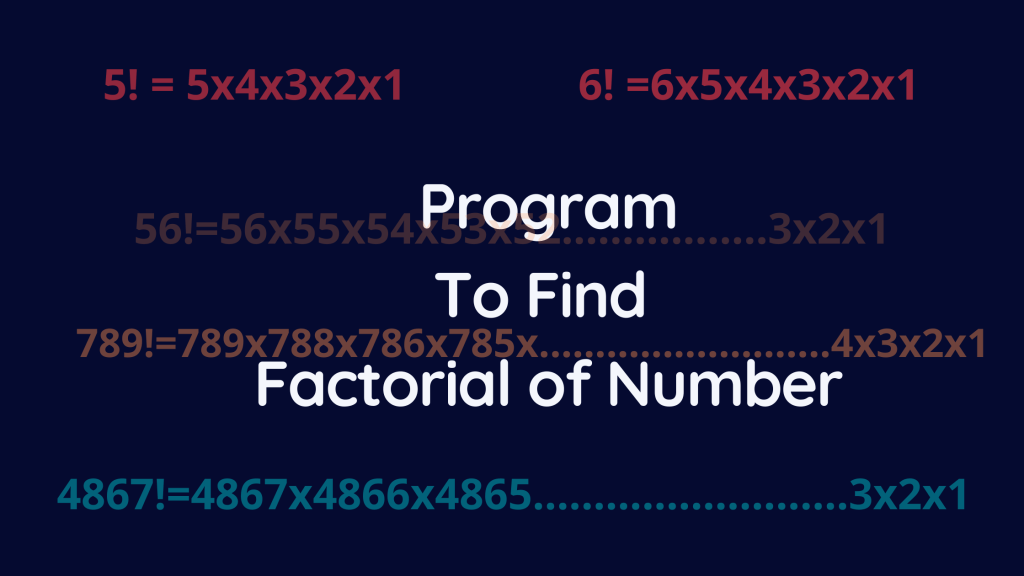# Python : Factorial program using for loopHello Friends,

So today in this article we are going to discuss about how to find factorial of any number in Python

Before discussing program lets discuss what is for loop in Python

What is for loop?

A for loop in Python is a control flow statement that is used to repeatedly execute a group of statements as long as the condition is satisfied.

Syntax

``for var in range(Starting number, ending number, optional number):``

where,

• var is variable name
• Starting number denotes where the range start
• Ending number denotes where the range ends
• Optional number is the optional step of increment or decrement (Default is +1 and -1)

Example of for loop

Print 1 to 5 numbers using for loop

``````for icounter in range(1,6):
print(icounter)``````

Python Program to find the factorial of a number in Python

1. To take input from the user
``num = int(input("Enter a number: "))``

Here we will set the value of factorial

``factorial = 1``

2. Check if the number is negative, positive or zero

``````if num < 0:
print("Sorry, factorial does not exist for negative numbers")``````

3. If the number equals to zero will print the factorial of 0 is 1

``````elif num == 0:
print("The factorial of 0 is 1")``````

4. If the number is positive. Here we are using for loop

``````else:
for iCounter in range(1,num + 1):
factorial = factorial*iCounter
print("The factorial of",num,"is",factorial)``````

Complete program

``````num = int(input("Enter a number: "))

factorial = 1

if num < 0:
print("Sorry, factorial does not exist for negative numbers")
elif num == 0:
print("The factorial of 0 is 1")
else:
for iCounter in range(1,num + 1):
factorial = factorial*iCounter
print("The factorial of",num,"is",factorial)``````

Output in visual studio code